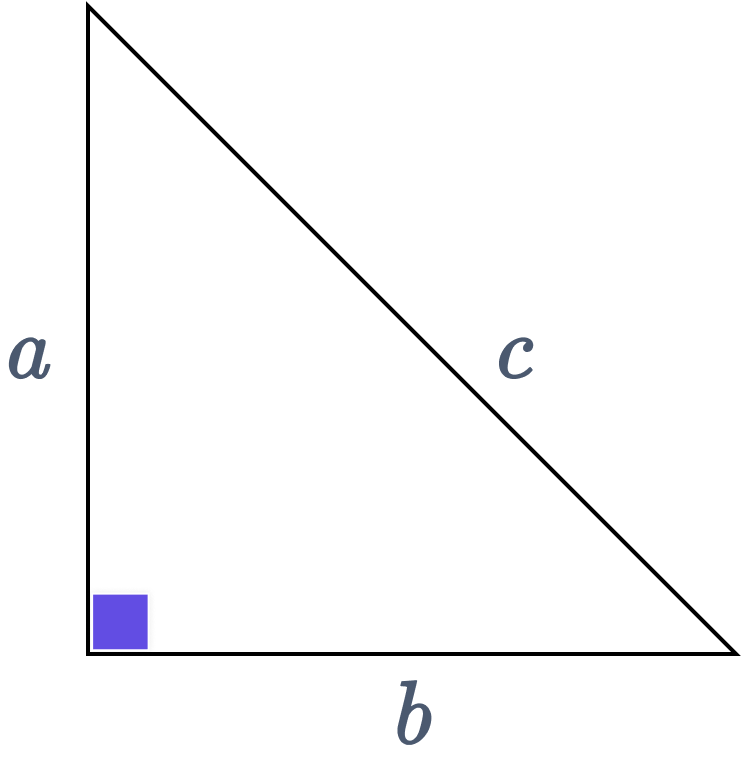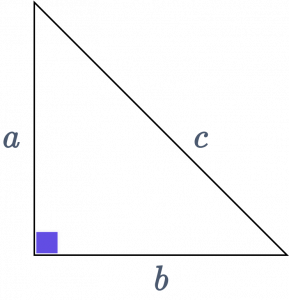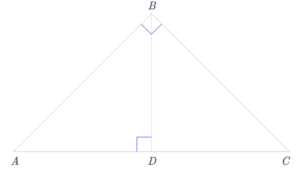# Pythagorean Theorem Calculator

The Pythagorean Theorem Calculator uses the Pythagorean theorem to determine the length of the third side in a right triangle based on the given lengths of the other two sides. By leveraging the power of The Mathematics Master online Pythagorean theorem calculator, these computations are performed quickly, providing users with immediate access to the value of the unknown side.$$a^2 + b^2 = c^2$$

## Pythagorean Theorem

The Pythagorean Theorem, commonly called Pythagoras’ Theorem, named after the Greek philosopher Pythagoras, is a fundamental relationship between a right triangle’s three sides. The Pythagorean theorem asserts that, given a right triangle, which is a triangle in which one of the angles is 90 degrees, the area of the square formed by the hypotenuse, the right triangle’s longest side, is equal to the sum of the areas of the squares formed by its other two sides:## Pythagorean Theorem Formula

If you know the lengths of any two other sides, you can use the Pythagorean Theorem formula for right triangles to get the length of the third side. See the following formulas for solutions derived from the Pythagorean Theorem:

$$Perpendicular^2 + Base^2 = Hypotenuse^2$$

(or)

$$a^2 + b^2 = c^2$$

$$a = \sqrt{c^2 – b^2}$$

$$b = \sqrt{c^2 – a^2}$$

$$c = \sqrt{a^2 + b^2}$$

## Derivation of Pythagorean Theorem Formula Using Similar TrianglesLet’s consider a right-angled triangle ABC, with the right angle at vertex B. We will draw a perpendicular line BD intersecting side AC at point D to prove the Pythagorean theorem. Using the concept of similar triangles, we can establish the proof as follows:

In triangles ABD and ACB:
Angle A in triangle ABD equals angle A in triangle ACB (common angle).
Angle ADB in triangle ABD equals angle ABC in triangle ACB (both are right angles).

Therefore, we can conclude that triangles ABD and ACB are similar by the AA (Angle-Angle) similarity criterion.

Similarly, we can prove that triangles BCD and ACB are also similar.

From the similarity of triangles ABD and ACB, we have the proportionality: $$\dfrac{AD}{AB} = \dfrac{AB}{AC}$$. By rearranging this equation, we obtain $$AD × AC = AB^2$$.

Similarly, from the similarity of triangles BCD and ACB, we have the proportionality: $$\dfrac{CD}{BC} = \dfrac{BC}{AC}$$. Rearranging this equation gives us $$CD × AC = BC^2$$.

Adding the two equations obtained in steps 3 and 4, we get $$AB^2 + BC^2 = (AD × AC) + (CD × AC)$$.

Simplifying the right-hand side of the equation, we have $$AB^2 + BC^2 = AC(AD + DC)$$.

Finally, recognizing that AD + DC equals AC (since AD + DC = AC), we can substitute it into the equation to obtain $$AB^2 + BC^2 = AC^2$$.

By the transitive property of equality, we have successfully proven the Pythagorean theorem: the sum of the squares of the lengths of the two shorter sides of a right-angled triangle equals the square of the hypotenuse length.

## How To Use Pythagorean Theorem

Example 1: Let a = 3 and b = 4

then we can determine the length of c as:

$$c = \sqrt{a^2 + b^2} = \sqrt{3^2+4^2} = \sqrt{25} = 5$$

We can calculate the length of any side of a triangle using the law of cosines which is a generalization of the Pythagorean theorem if we know the angles and lengths of the other two sides of the triangle. The law of cosines reduces to the Pythagorean equation if the angle between the opposite sides is a right angle.

Example 2: The sides of a triangle are 9, 40, and 41 units. Check if it has a right angle or not.

To determine if the triangle has a right angle, we can apply the Pythagorean theorem, which states that in a right triangle, the square of the length of the hypotenuse is equal to the sum of the squares of the other two sides.

Let’s assign the side lengths as follows:

Perpendicular (P) = 40 units
Base (B) = 9 units
Hypotenuse (H) = 41 units (since it is the longest side measure)

According to the Pythagorean theorem, we have:

$$LHS = P^2 + B^2$$
$$=> 40^2 + 9^2$$
=>1600 + 81
=>1681

$$RHS = H^2$$
$$=> 41^2$$
=> 1681
We can observe that the LHS (1681) equals the RHS (1681). LHS = RHS Therefore, since the equality holds, the angle opposite to the 41 units side will be a right angle.

## FAQs

What is the use of a special right triangle calculator?

Special right triangles have three defined interior angles and fixed side ratios. If one side is known in these right-angled triangles, we can find the value of the two missing sides using the special right triangle calculator. For example, the 45° – 45° – 90° triangle and the 30° – 60° – 90° triangle are other names for the two special right triangles.

What is the Pythagorean theorem calculator right triangle formula?

Pythagoras’ theorem asserts that: $$a^2 + b^2 = c^2$$ in a right triangle with cathetus a and b and with hypotenuse c. Take the square root of both sides to find the answer to the equation for c:

$$c = \sqrt{b^2 + a^2}$$. This Pythagorean theorem extension can be considered a “Hypotenuse Formula.”

What is the significance of the Pythagorean theorem?

The Pythagorean theorem has several practical applications. It can be used to determine the steepness of mountains. It helps calculate the distance between an observer and a point on the ground from a tower or a building when the observer is viewing the point from above.

What are the different methods of proving the Pythagorean theorem?

There exist various approaches to prove the Pythagorean theorem. Some of the commonly employed methods include:

• Using similar triangles
• Using differentials
• Algebraic proof
• Euclid’s proof